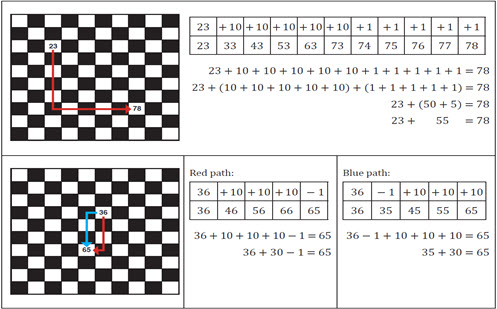Go to website

# reSolve: Addition: Chess: The Rook

This lesson engages students in investigating place value and the addition and subtraction of numbers by exploring computation on the number chart. Students analyse the moves of a rook chess piece and how the value of the numbers change as it moves. This builds into an exploration of how the number chart can be used as a tool to aid and model addition and subtraction using tens and ones. The lesson is outlined in detail including curriculum links, discussion points, extension activities and student handouts. This sequence is part of the reSolve: Mathematics by Inquiry program.

Year level(s) Year 3
Audience Teacher
Purpose Teaching resource
Teaching strategies and pedagogical approaches Mathematics investigation
Keywords addition, number charts, place value, subtraction

## Curriculum alignment

Curriculum connections Numeracy
Strand and focus Number, Build understanding, Apply understanding
Topics Addition and subtraction, Place value
AC: Mathematics (V9.0) content descriptions
AC9M3N03
Add and subtract two- and three-digit numbers using place value to partition, rearrange and regroup numbers to assist in calculations without a calculator

AC9M3A01
Recognise and explain the connection between addition and subtraction as inverse operations, apply to partition numbers and find unknown values in number sentences

AC9M3A02
Extend and apply knowledge of addition and subtraction facts to 20 to develop efficient mental strategies for computation with larger numbers without a calculator

Number and place value (P5)
Number patterns and algebraic thinking (P3)